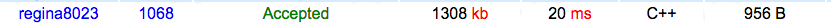# 【BZOJ 1068】 [SCOI2007]压缩

## 1068: [SCOI2007]压缩

Time Limit: 1 Sec  Memory Limit: 162 MB
Submit: 518  Solved: 331
[Submit][Status]

## Description## Sample Input

bcdcdcdcdxcdcdcdcd

12

## HINT

【限制】

100%的数据满足：1<=n<=50 100%的数据满足：1<=n<=50

f[l][r][k]表示i-j这段区间，是否放置M(k=1/0)的最短长度。注意此时l-1一定是有M的，或者l-1=0。

k可以是

1，1

1，0

0，1

0，0

#include <iostream>
#include <algorithm>
#include <cstring>
#include <cstdlib>
#include <cmath>
#include <cstring>
#include <cstdio>
#define inf 0x3f3f3f3f
using namespace std;
char s;
int f;
bool same(int l,int r)
{
int now=r-l+1;
if (now&1) return 0;
now/=2;
for (int i=l;i<l+now;i++)
if (s[i]!=s[i+now]) return 0;
return 1;
}
int dp(int l,int r,int k)
{
int now=r-l+1;
if (now==1)
{
if (k) return inf;
return f[l][r][k]=1;
}
if (f[l][r][k]<=100) return f[l][r][k];
if (k)
{
int mi=inf;
for (int i=l;i<r;i++)
{
mi=min(mi,dp(l,i,1)+1+dp(i+1,r,1));
mi=min(mi,dp(l,i,0)+1+dp(i+1,r,1));
mi=min(mi,dp(l,i,1)+1+dp(i+1,r,0));
mi=min(mi,dp(l,i,0)+1+dp(i+1,r,0));
}
return f[l][r][k]=mi;
}
if (same(l,r)) f[l][r][k]=dp(l,l+now/2-1,0)+1;
for (int i=l;i<r;i++)
f[l][r][k]=min(f[l][r][k],dp(l,i,0)+r-i);
return f[l][r][k];
}
int main()
{
scanf("%s",s+1);
int l=strlen(s+1);
memset(f,inf,sizeof(f));
printf("%d\n",min(dp(1,l,1),dp(1,l,0)));
return 0;
}©️2019 CSDN 皮肤主题: 技术黑板 设计师: CSDN官方博客